# Analytical NMR – Identification, Quantification (qNMR) and Mixture Analysis

NMR is best known for its use in structure determination. The chemical structure of a small molecule dictates a rational spectrum. This is because the electronic structure, the bedrock of chemistry, is what determines the chemical shift, while the number of nuclei determine the coupling and relative intensities. These same properties result in NMR being excellent for quantification and identification. The peaks in the spectrum will appear in predictable locations in the spectrum and their intensities will be proportional to the number of nuclei in the sample. In other words, the peaks are a chemical signature and the sample concentration is proportional to the intensity of the NMR signal detected. Sample concentrations and purities can be easily measured from known peaks once the proportionality constant is calibrated using a reference of known concentration. Using these peak positions and relative intensities, the compounds, mixtures and impurities in a sample can be identified or quantified.

Examples for these features of analytical NMR can be found here:

## Identification

NMR spectra can be used to identify compounds. Depending on the degree of specificity required, this can be a simple peaks comparison or can incorporate other tests related to peak splitting and integral intensities. Here we show a method for identification of ascorbic acid, which is a common vitamin sold on its own as well as in many dietary supplements.

The United States Pharmacopeia method for identification of ascorbic acid requires both FT-IR and a reducing sugar chemical test because neither has the specificity on its own. Here a single NMR spectrum is acquired and analysed with three different tests: peaks, multiplet, and nuclides count comparisons to quickly identify ascorbic acid. A similar set of tests is used for sodium ascorbate.

### Peaks Test

The peak positions in the spectrum are compared to a peaks list determined from a standard material. If the peaks match, then the sample passes the peaks comparison test. The peak positions are shown above the spectrum and range from 3.64 to 4.99 for this sample.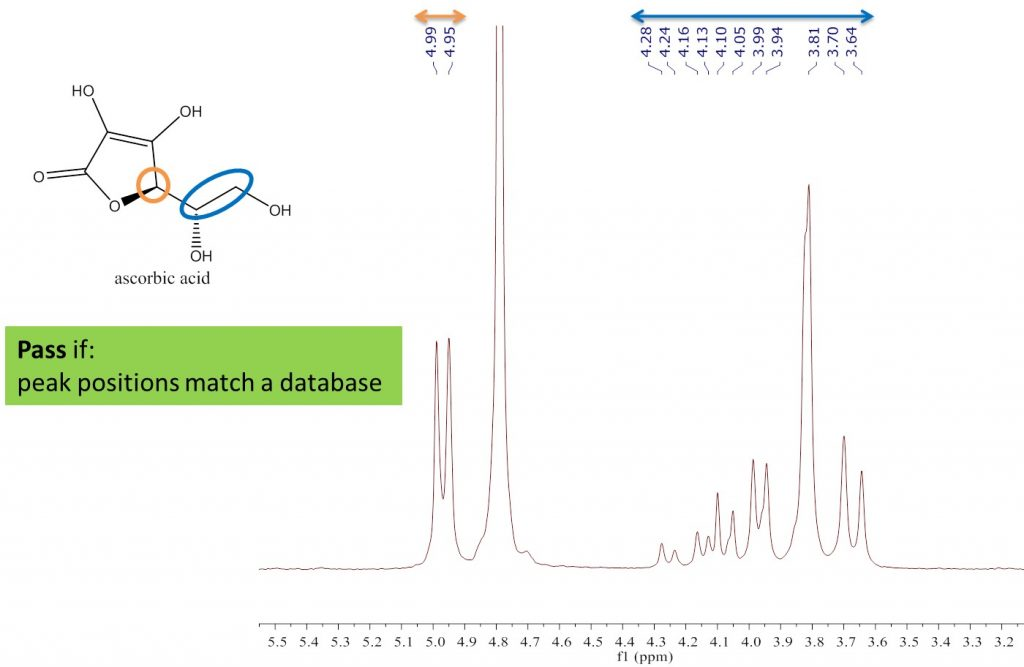Figure 1: Peak matching of the ID test.

### Multiplet test

The multiplet position and multiplicity of the peaks in the spectrum are compared to those determined from a standard material. If the two match, then the sample passes the peaks comparison test. The peak positions and multiplicity are shown in the boxes on the spectrum. Peak A is a doublet (d) at 4.96 ppm and Peak B is a multiplet (m) at 3.85 ppm.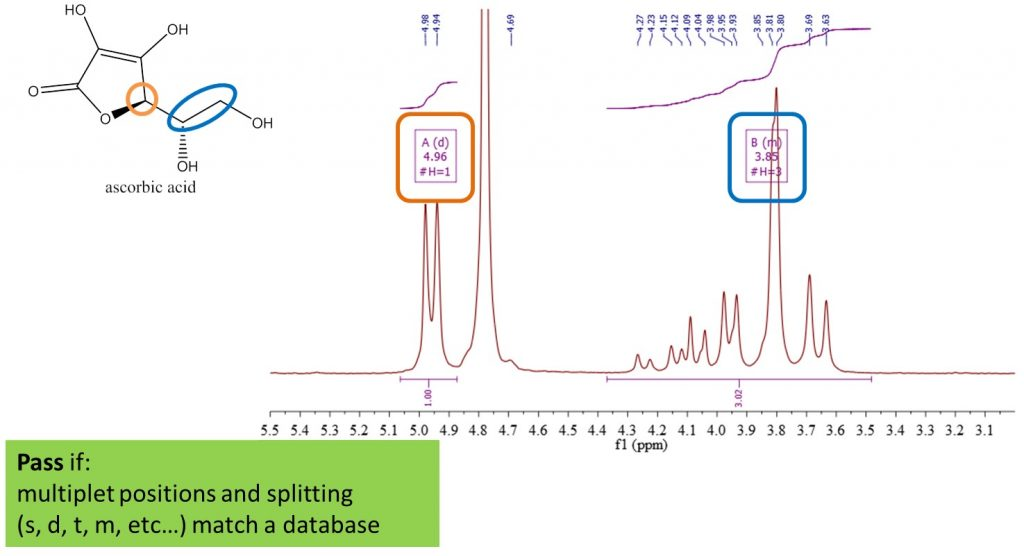Figure 2: Multiplet matching of the ID test.

The sample must past all three tests to be positively identified as ascorbic acid. This identification test was validated using the following tests for specificity and reproducibility.

 Test Category Result Positive identification Specificity 12 samples resulted in positive IDs Negative control Specificity 4 analytes not identified as ascorbic acid Repeatability Precision 6 standards measured in one day Intermediate Precision Precision 3 standards measured over three days Reproducibility (Ruggedness) Precision 6 standards measured in two labs

These results meet the UPS specifications for an identification method.

## Quantification – Internal Standard

Quantification using any analytical method is no more than calibrating an instrumental response with a known reference, and then calculating the concentration of an unknown sample from the measured instrument response. One method of calibrating the NMR spectrometer is with an internal standard. Both the sample and the reference are weighed out and co-dissolved into a single solution. The integral of the peak associated with the reference sample is used to calibrate the instrument response. One or more peaks associated with the sample of interest are then used to determine the sample concentration or purity. The purity can be calculated with the following equation.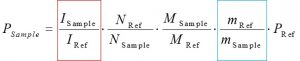Where:

• M =Molecular weight
• I = Integral
• N = Normalisation factor (the number of nuclei represented by the peak)
• m = Mass
• P = Purity
• Sample = designates the sample of interest
• Ref = Reference Standard

### Methylsulfonyl methane

When quantitative methods are validated there are standard requirements for accuracy, precision, range, and linearity over that range. Table 1 below shows general requirements for a Category I NMR method when measuring a drug substance (there are other specifications for finished products and impurities).

These requirements were tested by measuring the purity of one reference standard, methylsulfonylmethane (MSM), with another, maleic acid. Maleic acid is a common reference standard so this was used as the reference to measure the known purity of MSM (99.5%). A spectrum of the mixture in D2O is shown in Figure 3.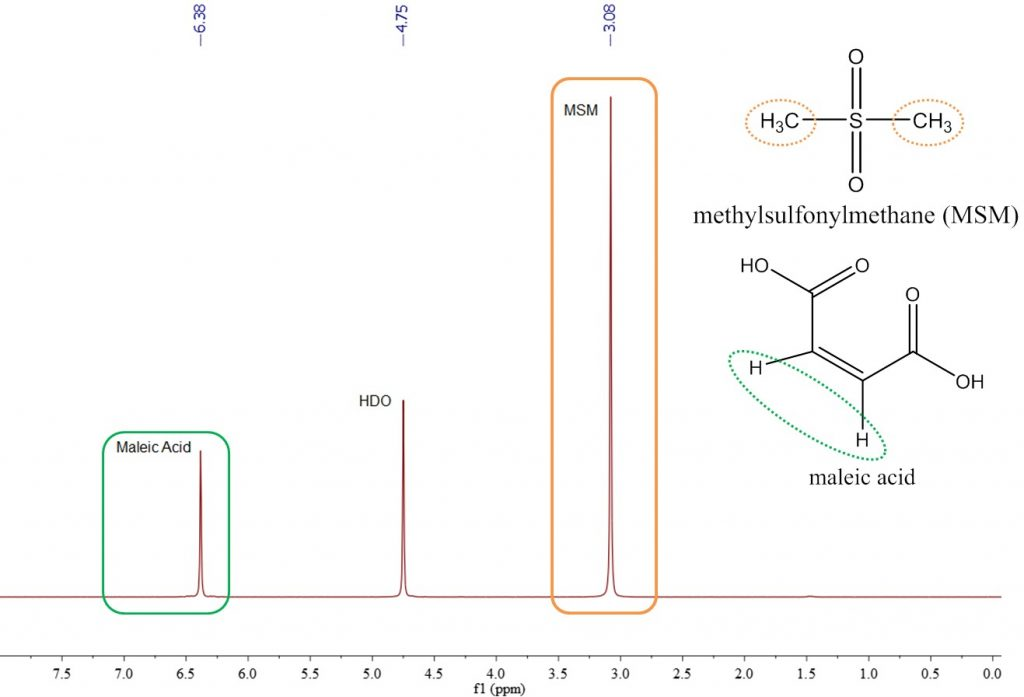Figure 3: Spectrum of MSM and maleic acid in D2O

Maleic acid and MSM were co-dissolved in D2O at a concentration of approximately 60 mg/mL. The maleic acid peak is a singlet, has two protons, and has a chemical shift of about 6.3 ppm. The methyl peak of MSM is a singlet representing six protons and located at 3 ppm. The purity was determined from these peaks to assess the accuracy and precision of this method.Figure 4: Purity for six independently prepared maleic acid and MSM solutions.

Figure 4 shows the purity measured by NMR for six independently prepared maleic acid and MSM solutions. The mean purity and standard deviation measured for MSM was 99.5 ±0.6%. This shows an accuracy within 2% and repeatability (precision) of less than 1%.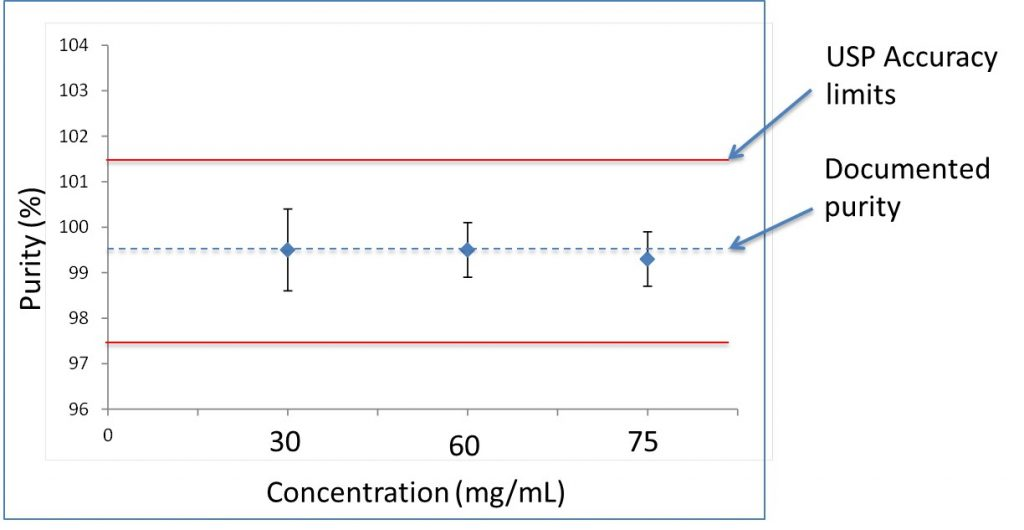Figure 5: The measured MSM purity for 30, 60 and 75 mg/mL (50-120 % of 60 mg/mL).

This experiment was repeated for 30 and 75 mg/mL (50-120% of 60 mg/mL). Over this range the accuracy and precision were 0.9, 0.6, and 0.6%, which is shown in Figure 5.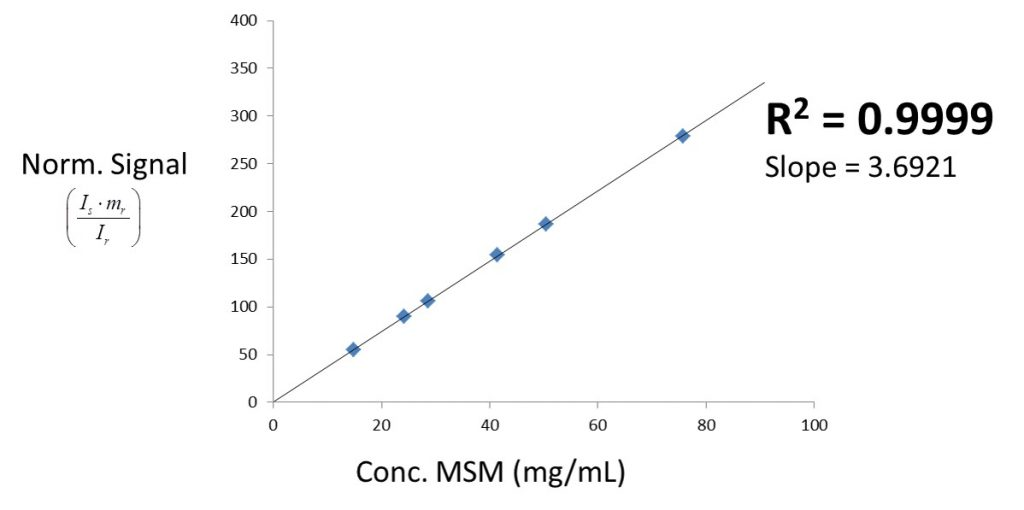Figure 6: Linearity check of signal versus concentration.

The linearity test between 15 mg/mL and 75 mg/mL is shown in Figure 4. A correlation coefficient (R2) of 0.9999 was determined, confirming highly linear behaviour between peak integral and concentration.

The results of these experiments are shown in Table 1. This method meets the accuracy, repeatability, range, and linearity requirements for a typical measurement of a drug substance. The uncertainty of these results is for the preparation and measurement of the samples. The error budget for weighing the samples is about 0.3 % or half of the total uncertainty.

 USP Specification Measured Accuracy 98-102 % 100 % Repeatability 1 % 0.6 % Range 80-120 % 50-125 % Linearity 0.995 0.999

USP <761> Nuclear Magnetic Resonance / Physical Tests

Table 1: USP requirements for accuracy, repeatability, range and linearity compared to performance of Spinsolve benchtop NMR.

## Mixtures Analysis

Common mixtures include solvent systems or block copolymers. NMR is an alternative to common techniques such as chromatography or titration, which can be time intensive and complicated techniques.

### Liquor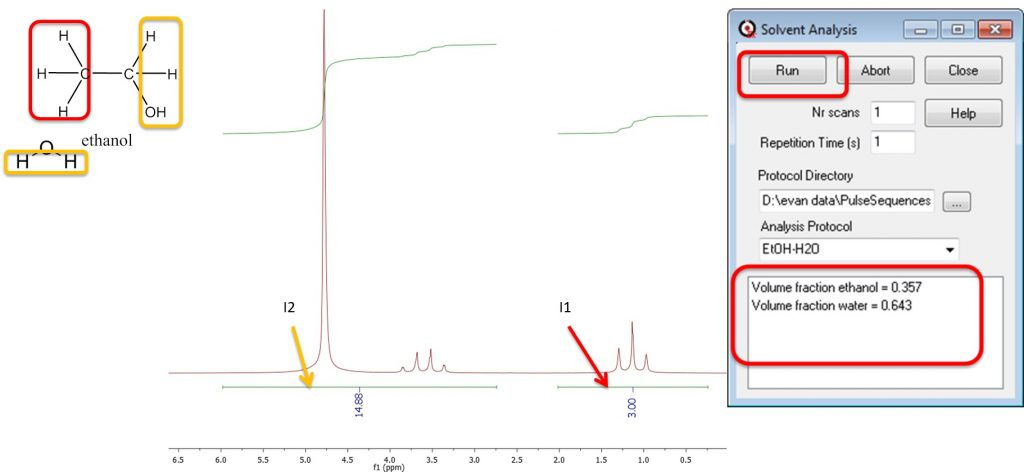Figure 7: Solvent mixture analysis of a Tequila sample.

Alcohol and water is a common solvent mixture. It contains one well resolved peak at about 1.2 ppm and two overlapping peaks between 2.7 and 6.0 ppm. The peak at 1 ppm is from the methyl protons (red box) of the ethanol and the other peaks are from the water protons and the alcohol and methylene protons from ethanol (yellow boxes). The integrals from the peaks can be represented by the following equations:

I1 = 3 EtOH; I2 = 2 H2O + 3 EtOH,

which can be transformed to calculate concentrations of each component using the following equations:

EtOH = I1/3; H2O = (I2 – 3 EtOH)/2.

The volume fraction or percent ethanol is calculated by converting from relative molar fractions to relative volumes using molecular weight and density.

This procedure and calculation was automated and then tested on tequila. After running the protocol we found that it contained 36% alcohol compared to 38% on the label.

### Poloxamer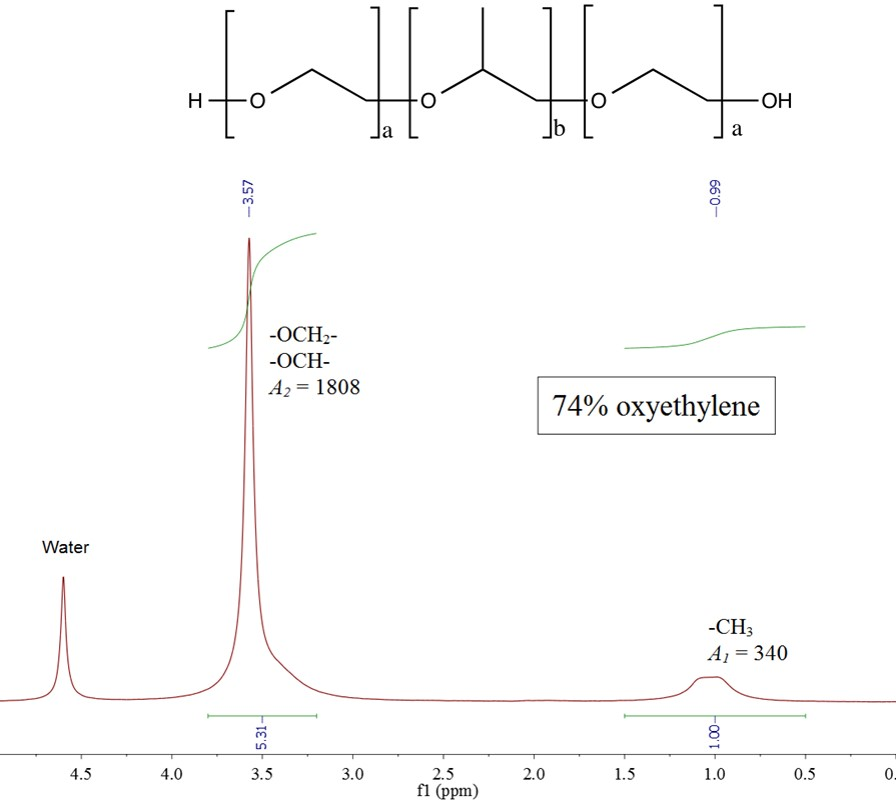Figure 8: Spectrum of a oxyethylene-oxypropylene block copolymer.

Poloxamers are oxyethylene-oxypropylene block copolymers commonly used as non-ionic surfactants. The ratio of oxyethylene to oxypropylene is a tuneable chemical property that is commonly determined by NMR spectroscopy. The peak at 1 ppm is from the propylene methyl and the peak at 3.5 ppm is from the polymer backbone. The weight percent oxyethylene is calculated using the following equations:The “7” in F127 means that the sample has approximately 70% oxyethylene and the NMR determined it to be 74%.

## Examples of 31P NMR

Protons are not the only nuclei that can be used for analytical measurements. Here phosphorous containing acids are used as examples of identification and quantifications using 31P as the observed nucleus.

### Identification

In methamphetamine production, red phosphorous is mixed with the precursor, ephedrine or pseudoephedrine. The phosphorus is converted to H3PO2 in the first stage of the reaction and then to H3PO3, and finally converting to H3PO4 at the end of the reaction. The amount of each acid provides an idea of what stage the synthesis was at and the potential yield.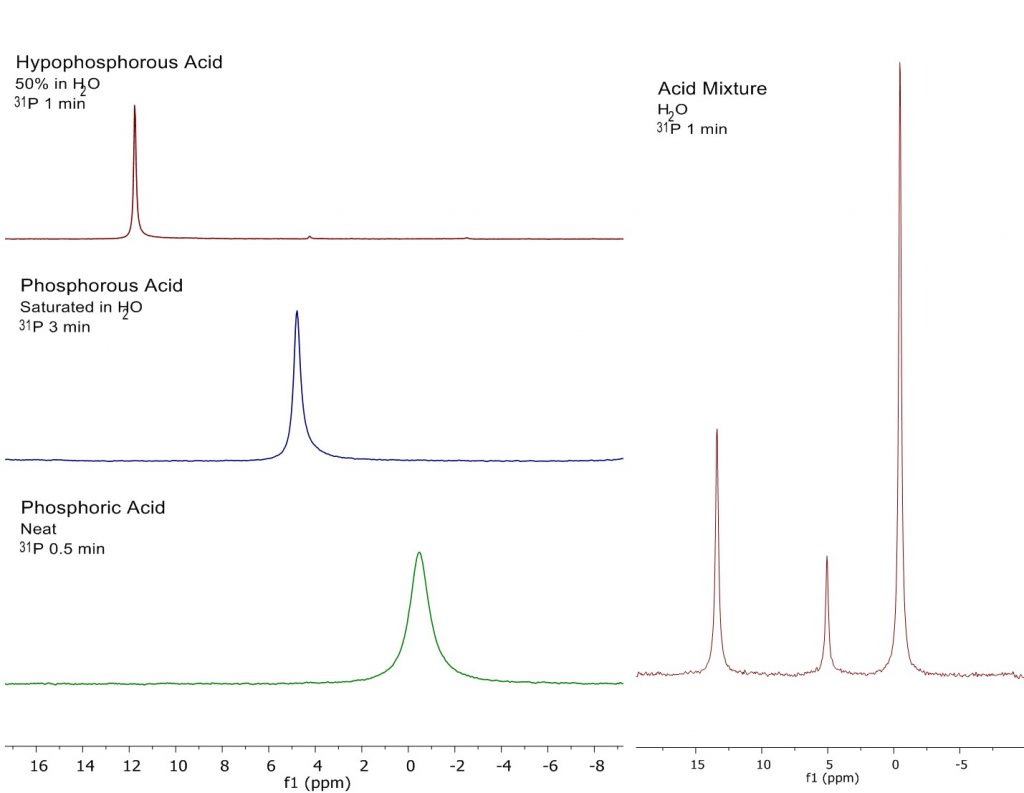Figure 9: 31P spectra of phosphoric, phosphorous and hypophosphorous acids (left) and mixture (right).

The chemical shift is used to identify the acid.

### Quantification

These strong acids are not stable conditions for internal standards therefore an external standard is used. The external standard method involves measuring a sample of known concentration and purity. The sample does not need to be the same as the sample of interest.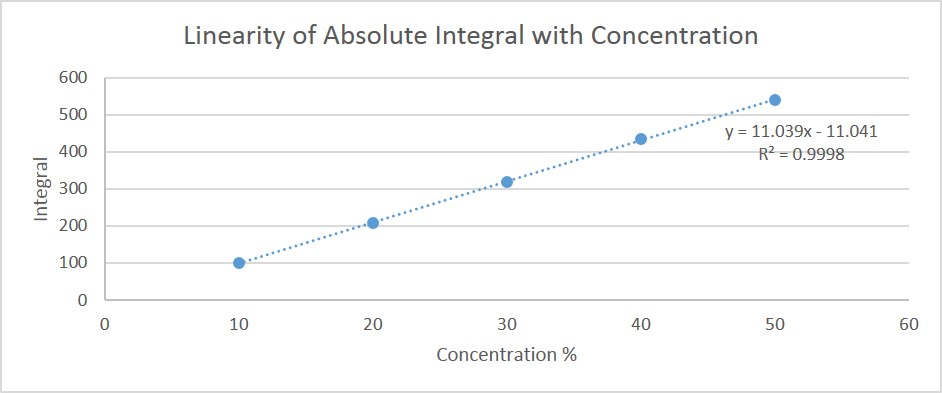Figure 10: Linearity of 31P signal with concentration.

Here you can see the linearity of the NMR integral with hypophosphorous concentration is >0.99 which means you can reliably estimate the concentration across this range.

The 30% hypophosphorous acid sample was used as an external standard for the samples listed in the table below.

 Sample Theoretical Concentration (w/w%) Calculated Concentration (w/w%) Hypophosphorous Acid 1 50 50.76 Hypophosphorous Acid 2 40 40.93 Hypophosphorous Acid 3 30 30.00 Hypophosphorous Acid 4 20 19.67 Hypophosphorous Acid 5 10 9.40 Hypophosphorous Acid Casework Sample 1 about 50* 52.33 Hypophosphorous Acid Casework Sample 2 about 30* 18.05

*Case work samples were estimated to be about 30% and 50% hypophosphorous acid using IR spectroscopy.

Table 2: Measurement of hypophosphorous acid concentration using external standard.

The calculated concentration agrees well with the theoretical concentration for the hypophosphorous acid samples.

### Related products

Spinsolve and Spinsolve Carbon benchtop NMR spectrometers